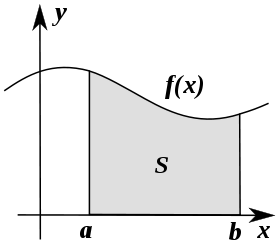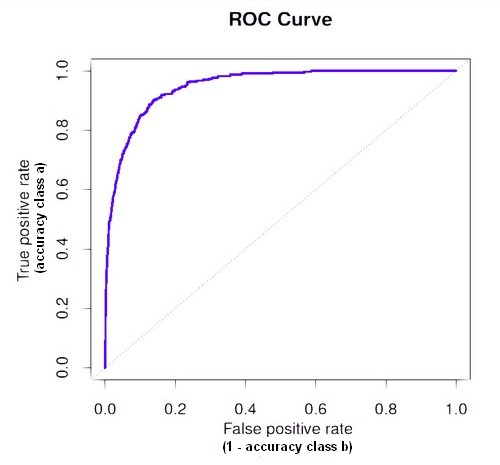# Machine Learning - Area under the curve (AUC)

The Area under the curve (AUC) is a performance metrics for a binary classifiers. By comparing the ROC curves with the area under the curve, or AUC, it captures the extent to which the curve is up in the Northwest corner. An higher AUC is good.

A score of 0.5 is no better than random guessing. 0.9 would be a very good model but a score of 0.9999 would be too good to be true and will indicate overfitting.

Recommended PagesCalculus - Integral

Integration can be thought of as measuring the area under a curve, defined by f(x), between two points (here a and b). Riemann integral was the first rigorous definition of the integral of a function...Statistics - ROC Plot and Area under the curve (AUC)

The Area Under Curve (AUC) metric measures the performance of a binary classification. In a regression classification for a two-class problem using a probability algorithm, you will capture the probability...